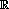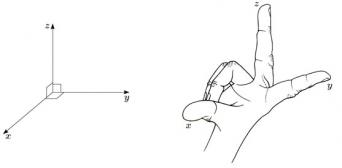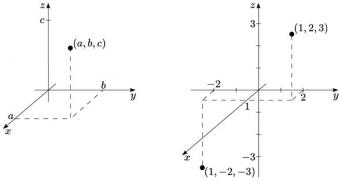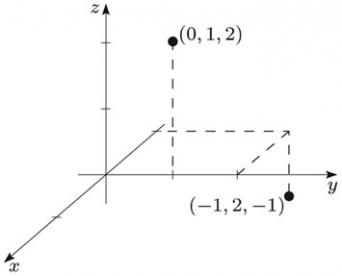Vectors and conics

This free course is available to start right now. Review the full course description and key learning outcomes and create an account and enrol if you want a free statement of participation.

Free course

1.6 Points, planes, lines and distances in three-dimensional Euclidean space

We now study three-dimensional space,3. This is a space with which you are familiar, of course, as ‘the real world’ is a three-dimensional space.

We define a coordinate system in three-dimensional space via three mutually perpendicular axes. Mutually perpendicular means that any two of the axes are at right angles to each other.

First, we choose a point O as the origin, and then we choose an x-axis and a y-axis at right angles to each other, as described earlier.

Next, we draw a third line through the origin, perpendicular both to the x-axis and to the y-axis; this line is called the z-axis. We choose the positive direction of the z-axis such that the x-, y- and z-axes form a so-called right-handed system of axes. This means that if you hold the thumb and first and second fingers of your right hand at right angles to each other, and label them x, y and z (in that order), you can turn your hand in such a way that your fingers point in the positive directions of the corresponding axes.

Finally, we choose a unit of distance along each axis.We have seen already that there is a one-one correspondence between the points of the plane and the ordered pairs (a, b) of real numbers. Similarly, there is a one-one correspondence between the points of three-dimensional space and the ordered triples (a, b, c) of real numbers. The point with coordinates (a, b, c) is reached from the origin by moving a distance a in the direction of the x-axis, a distance b in the direction of the y-axis, and a distance c in the direction of the z-axis.

For instance, the points with coordinates (1, 2, 3) and (1, −2, −3) are as shown in the diagram below.It may help you to visualise axes and coordinates in three dimensions by thinking of the corner of a room, with the x- and y-axes along the foot of the two walls, and the z-axis along the vertical join of the walls. To become familiar with this notation, choose axes as above for the room you are in, and determine the approximate coordinates of the corners of the room (using appropriate units of distance).Example 6

On a single diagram, sketch the x-, y- and z-axes and the points with coordinates (−1, 2, −1) and (0, 1, 2).In view of the one-one correspondence between points in three-dimensional space and ordered triples of real numbers, often we do not distinguish between the points and their representation as ordered triples; thus we simply write (a, b, c) to denote the point represented by this triple.

We call three-dimensional space, together with an origin and a set of x-, y- and z-axes, three-dimensional Euclidean space.

M208_1